## Wednesday, 7 September 2016

### The Generalized Egg Drop Problem

The original puzzle goes something like this: You have some identical eggs and a 100-storey building. You do not know what is the highest floor one can drop an egg from without breaking it, but for some reason you need to find out. What is the minimum number of drops that would guarantee an answer for you if can use only two eggs for testing?

In order to be sure that you've found the maximum safe height you need to have tested the next floor above: the minimum height required to break an egg. If you had only one egg available for testing, you'd have no choice but to start at the first floor and move up one at a time until you broke the egg. But with a second egg, you can skip some floors, using the first egg to bracket the solution. We're trying to minimize the number of times we'd have to drop the second egg one floor at a time. Supposing that we have a maximum number of available drops, d, regardless of whether we break the eggs or not, then each time we drop the first egg without breaking it, that's one less drop available for the second egg. So the most efficient procedure is to skip to the dth floor first. If it doesn't break, you go up by another d-1 floors. It it still doesn't break, you go up d-2, d-3, etc. until it does break (or you run out of floors). When the first egg finally does break, you go back to the one floor above the last floor that didn't break the first egg and test the second egg. You keep going up one at a time until you break the second one (or it doesn't break on the floor below the one that broke the first egg, in which case you know that you stumbled upon the minimum height to break an egg using your first egg). Mathematically, the tallest building we can test with d drops and 2 available eggs is given by the summation: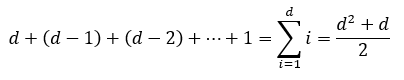So for the case of a building with 100 floors, we solve: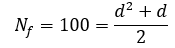This quadratic equation has two real roots, but we can ignore the negative root because it has no physical meaning for our problem. The positive real root is: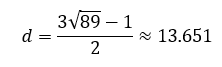A partial drop has no physical meaning either, so we have to round up to the next whole number, in this case 14. In other words, for a 100-floor building and 2 eggs, the minimum number of drops that will guarantee an answer is 14. You start by dropping the first egg on the 14th floor. If it doesn't break, you go up another 13 (to 27), then 12 (to 39), then 11 (to 50) etc. until it breaks, the test one floor at a time with the second egg.

Here are two examples of how the tests could go on our 100-storey building: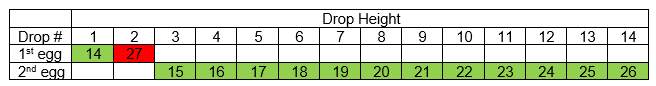Example of the optimal test procedure on a 100-floor building when only 2 eggs are available. Solution = 26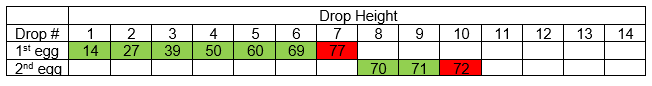Example of the optimal test procedure on a 100-floor building when only 2 eggs are available. Solution = 71

We can generalize the problem to any number of eggs, Ne. We don't know how many drops we will need, but if we specify a maximum of d drops, then hopefully we can solve for the maximum height, Nf of a building for which we are guaranteed be able to find a solution.

Now we don't know what Nf is yet, but let's pretend that we do already know how to find Nf for any numbers d drops and Ne eggs. We at least know that Nf is a function of d and Ne, which we can express mathematically as: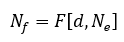What happens if we're told that we get one more egg and one more drop? Now the total number of floors we could test is F[d+1,Ne+1]. With our extra egg we'd like to bracket the solution such that if it breaks on our first drop we're left with only F[d,Ne] floors. So if we optimize our search procedure, when we're given one more egg and one more drop to start out with, there are two possible outcomes on the first drop:
1. We start out with F[d+1,Ne+1] floor to test. The first egg breaks on the first drop. We have Ne eggs and d drops remaining to find the maximum safe drop height from the remaining F[d,Ne] floors. We're pretending that we already know what the function F[d,Ne] is.
2. The first egg doesn't break on the first drop. Now we still have (Ne+1) eggs and d drops remaining for some number F[d,Ne+1] floors above.
This gives us a recursive relation that can be expressed mathematically as: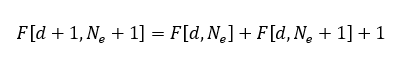or, equivalently: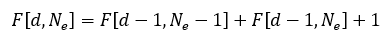Now as I said before, we still don't know what the general form of F[d,Ne] is, but we did already figured out the general form for when Ne = 2.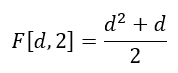We also know that when Ne = 1 we have no choice but to start at the bottom and go up one floor at a time until it breaks. The maximum number of floors we can test is equal to the number of times we can drop the egg.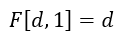There's also a simple boundary condition that will help us. If Ne = d, there can be no further benefit to adding another egg, because even if we broke an egg every time, we'd reach our limit on the number of drops first. This gives us a condition that can be expressed as: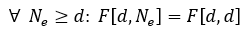We now have more than enough information to use the recursive relation and figure out how many floors could be tested with any number of eggs or drops. I've shown the first few steps below: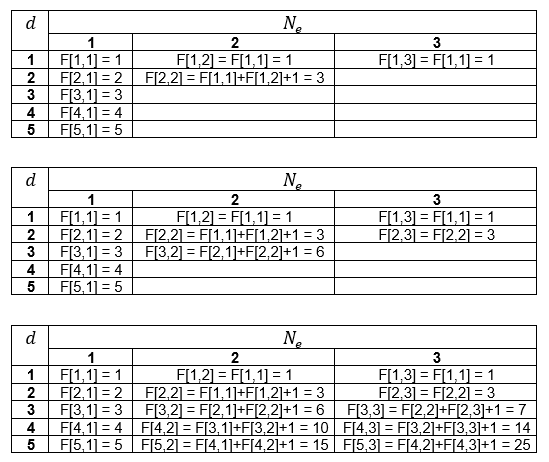Now if you use a spreadsheet it is fairly easy to automate these recursive calculations and create a large table of values, such as the one I made here: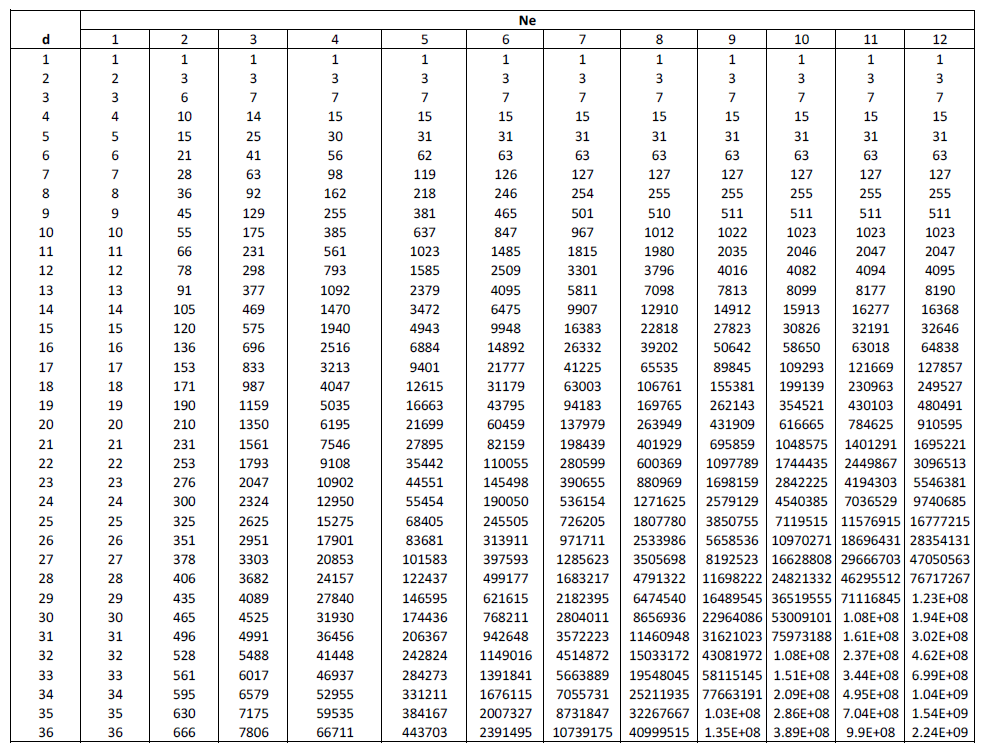Now we could stop here. Theoretically, we can produce a table of values of maximum building heights Nf for any numbers Ne eggs and d drops, though we have to do it by repeatedly adding some of our previous function evaluations together. If we already know the height of the building and the number of eggs available for testing, then we can go down the column for corresponding value of Ne until we reach the first value of Nf that is equal to or greater than the number of floors in our building. Then we go left across the row and read the value d. This will be the minimum number drops required to guarantee we find a solution to the maximum safe drop height in our building of Nf floors.

But, of course, we won't stop there. We still don't know how to express the function F[d,Ne], we just defined a recursive relation that allowed us to evaluate it anyway.

Using some optimal search algorithm there is one unique path to every possible solution within the building of Nf floors. If we have one egg, there are Nf = d paths, all of which involve breaking one egg. Each solution path represents a combination, a way to select 1 floor from d floors. One broken egg out of d floors. Using the binomial coefficient, we can express the number of ways of choosing 1 floor out of d floors as C(d,1) = d.

When we have two eggs, the maximum is (d)(d+1)/2 floors, or (d)(d+1)/2 unique solution paths. Only one egg breaks for some solution paths, while 2 eggs break for other solution paths. The total, (d)(d+1)/2, is equal to the sum of the one-egg paths plus the two-egg paths. Each one-egg path is a way of selecting one egg-breaking floor from d egg drops. C(d,1). Each two-egg path is a way of selecting two egg-breaking floors from d egg drops. C(d,2). So the total number of paths is: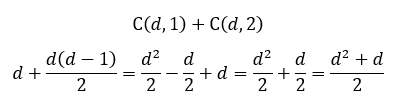Maybe you see where this is going. We haven't proven anything yet, but it looks like we can come up with a conjecture. Let us propose that for three eggs and d drops, the number of unique solution paths is equal to the number of paths where one egg breaks plus the number of paths where two eggs break plus the number of paths where three eggs break. In other words, C(d,1) + C(d,2) + C(d,3). Furthermore, let us propose that for Ne eggs and d drops, the number of unique solution paths is: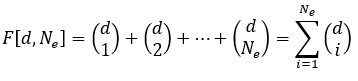Proposed general equation for the maximum number of floors that can be tested with d drops and Ne eggs.

Now all that's left to do is to prove the conjecture. I will use mathematical induction. We already know the conjecture is true for all d (greater than or equal to one) when Ne = 1 or 2. Next we assume that it is also true for any natural numbers d and Ne. If we can prove that the relation still holds true for d+1 and Ne+1, then the conjecture is true for all natural numbers. Starting with the recursive relation: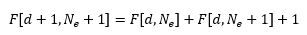Substituting the proposed general equation: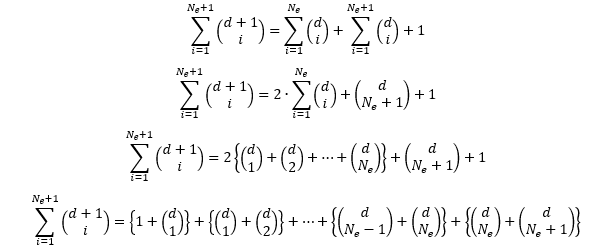Applying Pascal's Rule: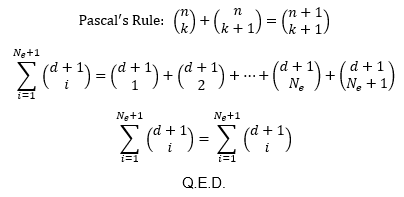So the conjecture has been proven. The maximum height of a building that can be checked using d drops and Ne eggs is Nf floors tall, where Nf is: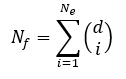Oh but we're still not done. The way the original problem was framed, we already know the height of the building and we're looking for the number of drops required. Unfortunately, there appears to be no general solution for that because it means having to solve for the positive real root of a polynomial with degree Ne. Apart from using a table as I described earlier, you could guess a value d, calculate Nf, and keep trying new guesses until you find the minimum value of d that gives Nf greater than or equal to the height of the building. But while we can't come up with a general solution for the minimum number of drops as a function of Nf and Ne, we can solve for a few cases. The cases Ne = 1 and Ne = 2 are easily done by hand. Closed-form solutions for the cubic and quartic functions exist, so the cases of Ne = 3 and Ne = 4 can also be solved analytically (though I definitely wouldn't attempt solving Ne = 4 by hand!). For Ne > 4, analytical solutions do not exist, with the exception of one special case. Here are the solutions for Ne = 1 to 4.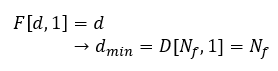Minimum number of drops when 1 egg is available for testing.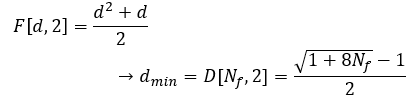Minimum number of drops when 2 eggs are available for testing.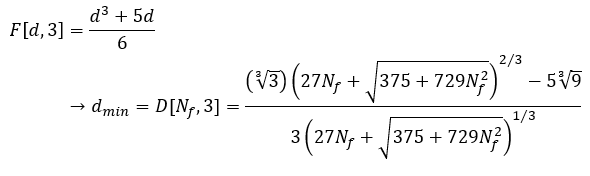Minimum number of drops when 3 eggs are available for testing.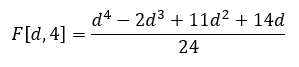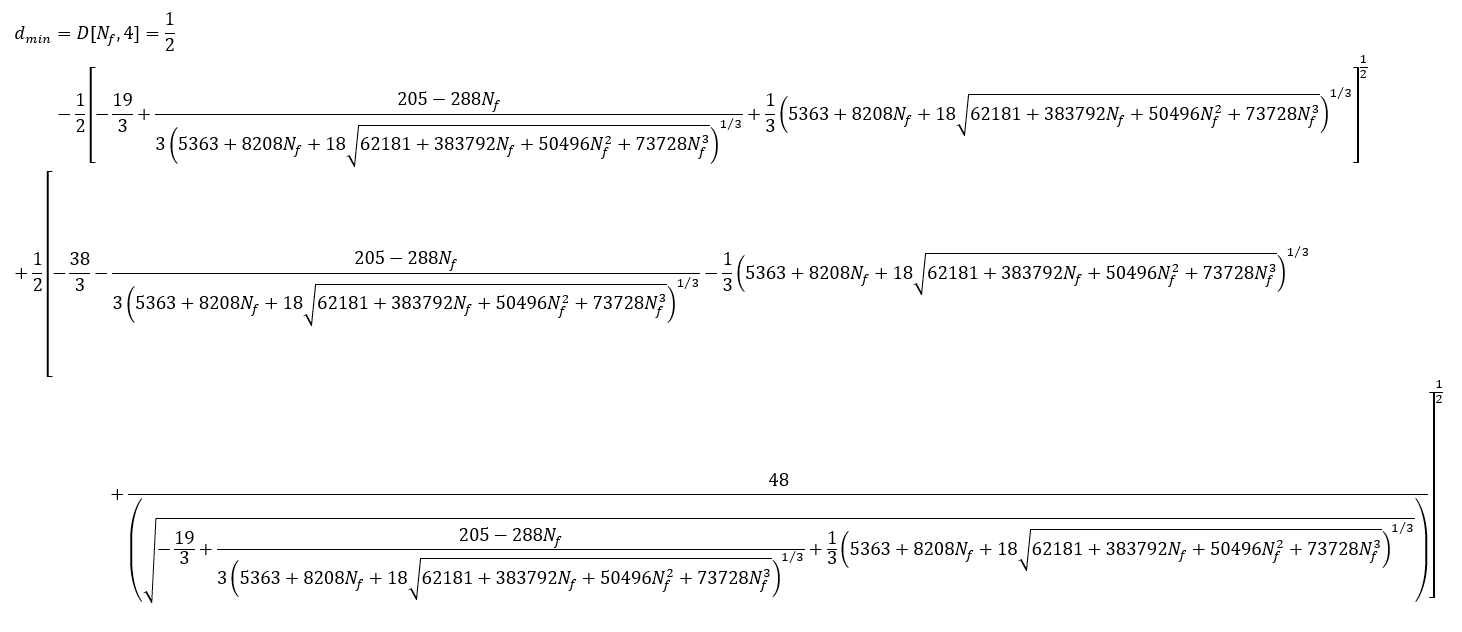Minimum number of drops when 4 eggs are available for testing.

Now I mentioned there was special case that could also be solved. That is the case of "many" eggs, that is, when Ne is at least equal to d. In this case: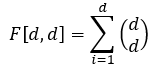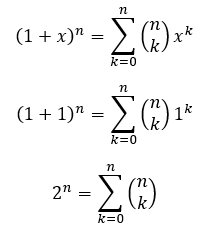And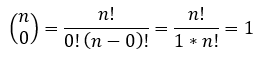Therefore,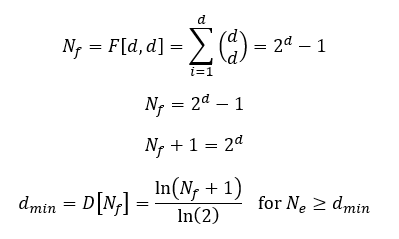Minimum number of drops when there are "many" eggs available for testing.

Finally. After all of that math, here's is a table and some graphs of the minimum number of drops required to find the maximum safe drop height in a building with Nf floors and with Ne eggs available for testing.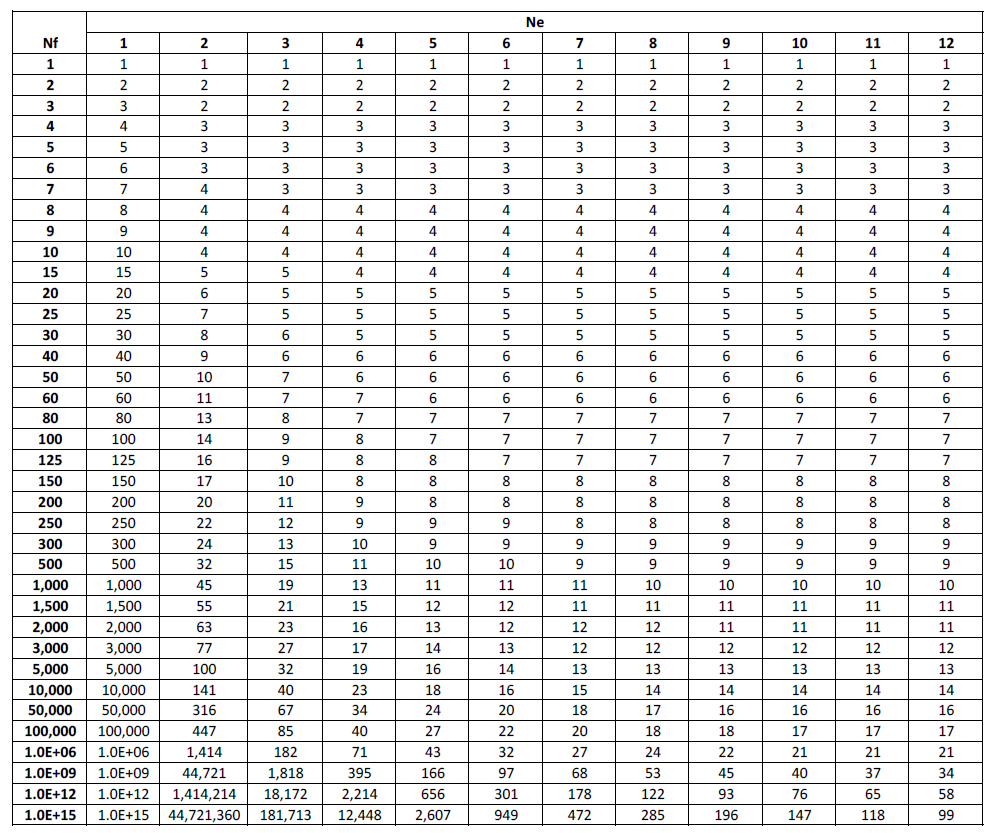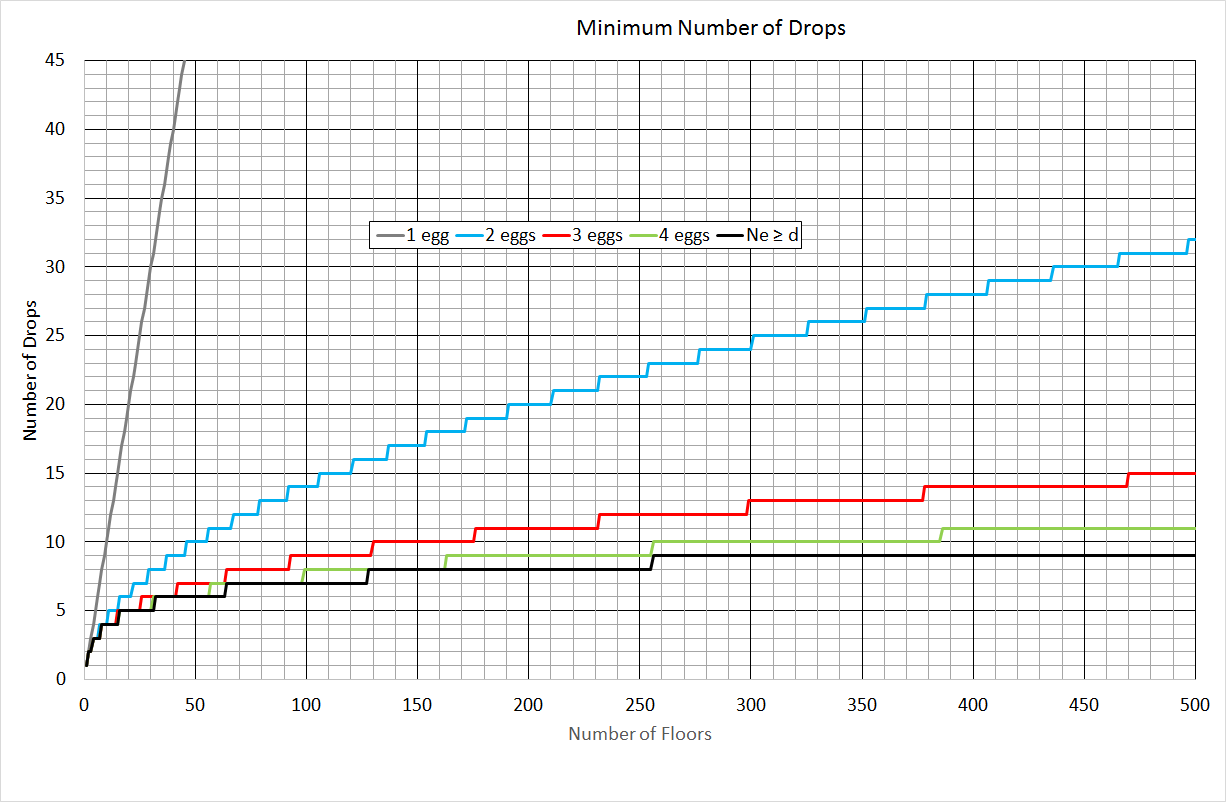You can see that two eggs is a massive improvement over one egg. Adding a third egg is pretty significant as well. With 4 eggs we have a pretty good approximation of the "many" eggs case, even in a hypothetical building with 500 floors (11 drops vs. 9 drops).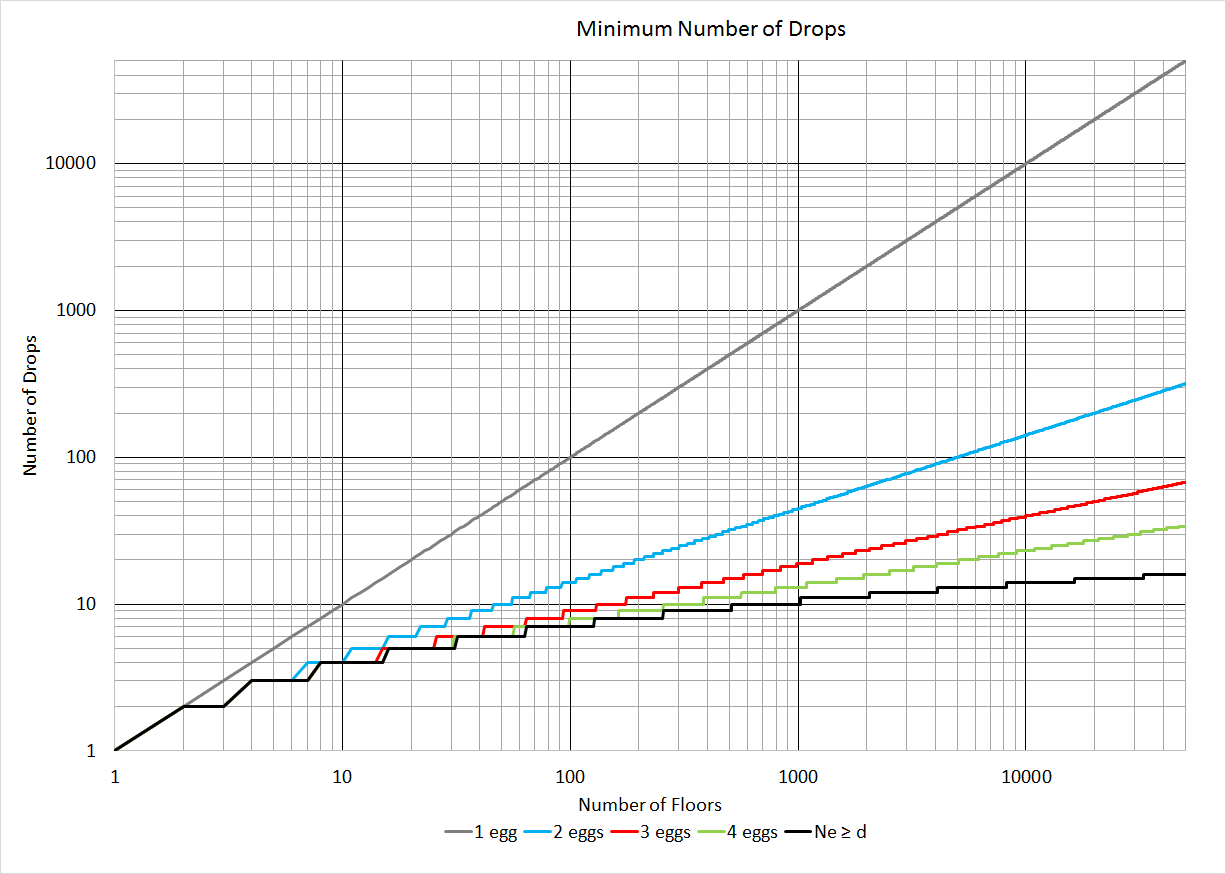In this graph I changed the axes to logarithmic scale and went all the way to 50,000 floors. I think it's a little easier to see how closely each of the D[Nf,Ne] functions approximate the best case (when Ne > d) at low values of Nf, but we also see that 4 eggs doesn't approximate "many" eggs very well beyond 500 floors.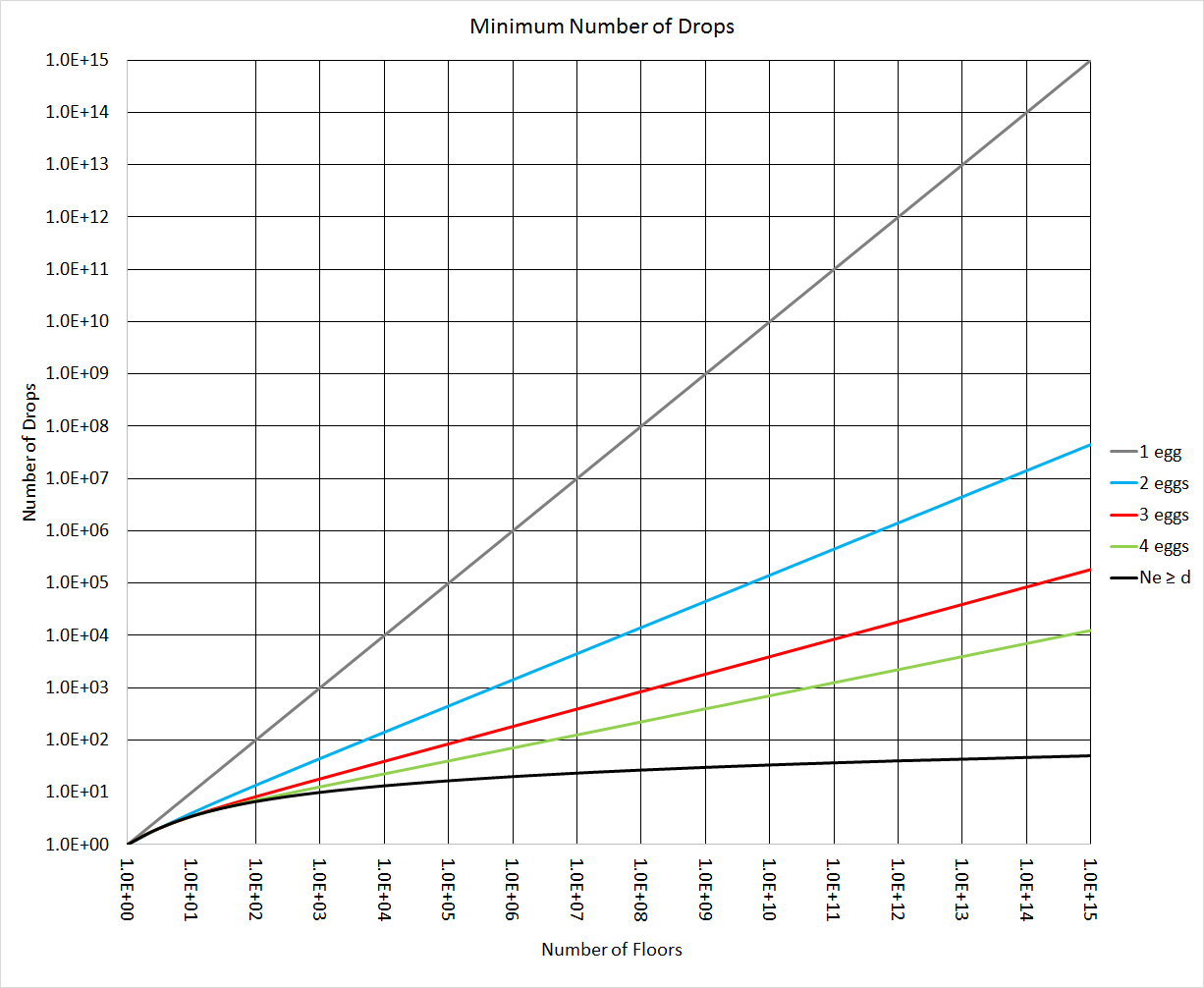In this final graph I approximated d by ignoring the steps in the function (i.e. I treated d as if it were defined for a continuum rather than just the natural numbers). That allowed me to go to an insanely high number of floors (1.0E15 = 1 quadrillion, or 1 followed by 15 zeros). At this scale you can see a significant difference between 4 eggs and "many" eggs for large values of Nf. With 30 eggs and 30 drops you could test a building with one billion floors. But if only 4 eggs were available you'd need to allow for 395 drops.

1.2.